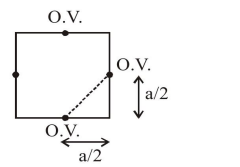# An element crystallises in a face-centred cubic (fcc) unit cell with cell edge a. The distance

Question:

An element crystallises in a face-centred cubic (fcc) unit cell with cell edge a. The distance between the centres of two nearest octahedral voids in the crystal lattice is

1. $\mathrm{a}$

2. $\sqrt{2} a$

3. $\frac{a}{\sqrt{2}}$

4. $\frac{\mathrm{a}}{2}$

Correct Option: 3,

Solution:distance between nearest octahedral voids(O.V.)

$=\sqrt{\left(\frac{\mathrm{a}}{2}\right)^{2}+\left(\frac{\mathrm{a}}{2}\right)^{2}} \quad \Rightarrow=\frac{\mathrm{a}}{\sqrt{2}}$